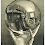## Thursday, November 19, 2009

### I Hope He Can Afford It

A man is buying a gold ring set with stones for his wife on her birthday. A ring set with 4 amethysts and 1 diamond comes to US\$2,000. One with 3 emeralds, 1 amethyst and 1 diamond would be US\$1,400. And one set with 2 rubies and 1 diamond would cost US\$3,000. Being a thoughtful husband, he choses a ring with 4 stones, each representing one of their 4 children.

As their children are named Andy, David, Ellen, and Richard, how much in US\$ will the ring containing 1 amethyst, 1 diamond, 1 emerald, and 1 ruby cost him?

1.I get US\$2,300:

1)4a + 1d = 2000

2)3e + 1a + 1d = 1400

3)2r + 1d = 3000
______________________

1a + 1d + 1e + 1r = x

(*6)

--->

6a + 6d + 6e + 6r = 6x

(- 3*eq. 3)

--->

6a + 3d + 6e = 6x - 9000

(- 2* eq. 2)

--->

4a + d = 6x - 11800

(- eq. 1)

--->

0 = 6x - 13800

--->

13800 = 6x

--->

x = 2,300

2.I got the same answer, but with way less math and mostly lucky guessing.

I took the last equation: 2 rubies + 1 diamond = 3,000 and guessed, "What if the rubies are \$1K each, and the diamonds are, as well?"

Took it from there to conclude that if the rubies and diamonds are each \$1K, then the first equation 4a + d = 2000 meant that amethysts cost \$250, and finally plugging that all into the second equation to figure emeralds cost \$50.

250 + 1,000 + 1,000 + 50 = 2,300

3.This comment has been removed by the author.

4.To solve this by simultaneous equations , we need 4 equations since we have 4 variables,
Oudeis created the 4 equation, since it was not readily given in the question.

Tabitha got it the easy way, guessing, I did the same thing, but started with very low values.
www.guessthelogo.blogspot.com

5.Interesting note though, guessthelogo:
We actually have 5 unknowns and 4 variables (note the "x" in the equation I start with), so none of these gems have a definite value. What I mean by that is: There are multiple solutions for the values of the gems themselves; only their sum is definite.

I'll illustrate by solving the equation set for "a", then providing two distinct solutions:

1)4a + 1d = 2000

2)3e + 1a + 1d = 1400

3)2r + 1d = 3000

Solve in terms of a:
d = 2000 - 4a

r = 1500 - d/2
= 1500 - 1000 + 2a
= 500 + 2a

e = (1400 - a - d)/3
= 1400/3 - a/3 - (2000 - 4a)/3
= -600/3 + a
= -200 + a

Solution # 1:
let a = 250
d = 1000
r = 1000
e = 50

(Verify:
1: 4a + d = 1000 + 1000 = 2000
2: 3e + a + d = 150 + 250 + 1000 = 1400
3: 2r + d = 2000 + 1000 = 3000)

Solution # 2:
let a = 300
d = 800
r = 1100
e = 100

(Verify:
1: 4a + d = 1200 + 800 = 2000
2: 3e + a + d = 300 + 300 + 800 = 1400
3: 2r + d = 2200 + 800 = 3000)

6.It would seem then, that the gold band is free.

I will take 20 without any stones please.

Leave your answer or, if you want to post a question of your own, send me an e-mail. Look in the about section to find my e-mail address. If it's new, I'll post it soon.

Please don't leave spam or 'Awesome blog, come visit mine' messages. I'll delete them soon after.

Enter your Email and join hundreds of others who get their Question of the Day sent right to their mailbox

The Lamplight Manor Puzz 3-D